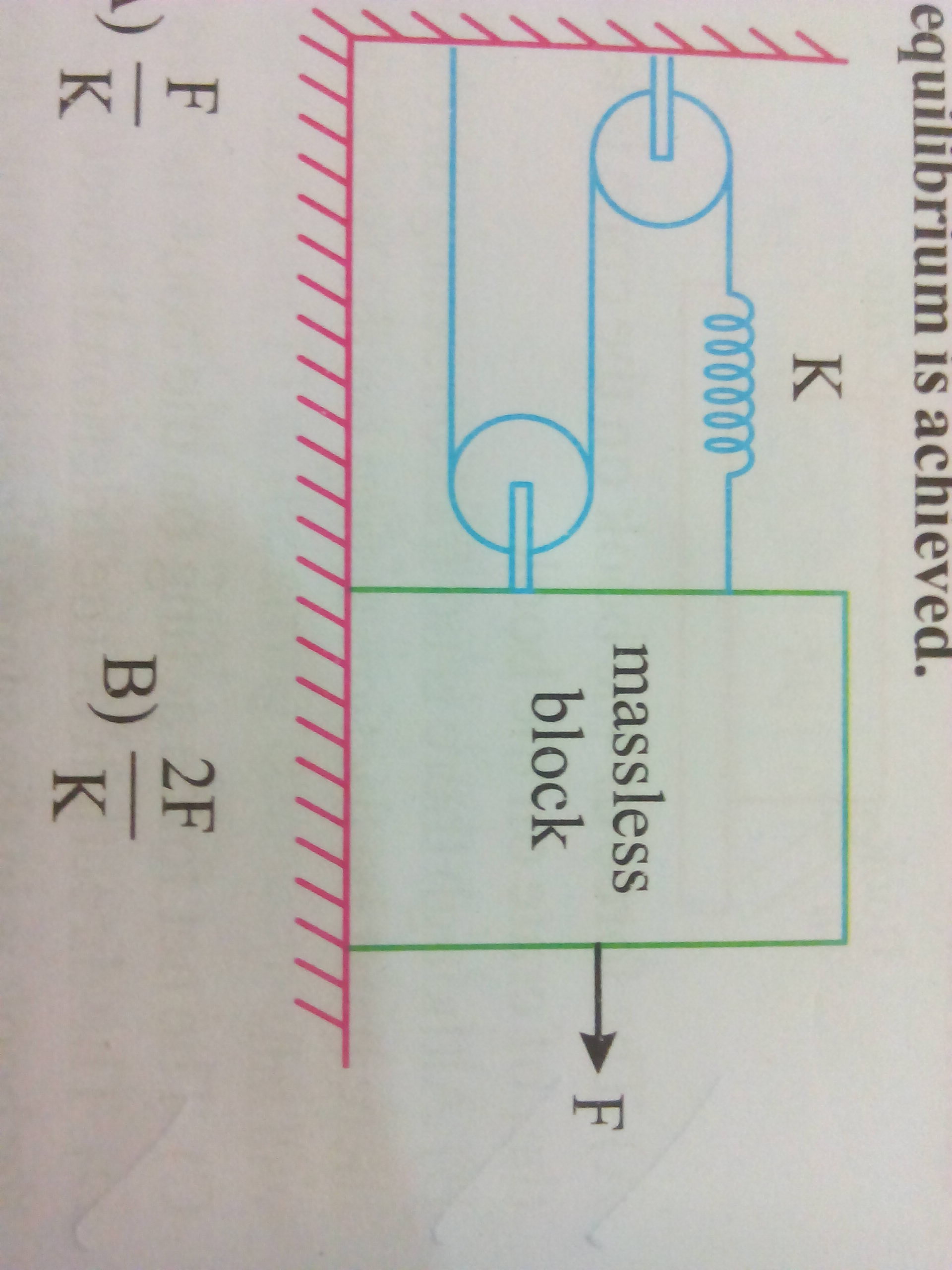# Equivalent spring constant?well sorry,the image should be horizontal.Note by A Former Brilliant Member
4 years, 5 months ago

This discussion board is a place to discuss our Daily Challenges and the math and science related to those challenges. Explanations are more than just a solution — they should explain the steps and thinking strategies that you used to obtain the solution. Comments should further the discussion of math and science.

When posting on Brilliant:

• Use the emojis to react to an explanation, whether you're congratulating a job well done , or just really confused .
• Ask specific questions about the challenge or the steps in somebody's explanation. Well-posed questions can add a lot to the discussion, but posting "I don't understand!" doesn't help anyone.
• Try to contribute something new to the discussion, whether it is an extension, generalization or other idea related to the challenge.

MarkdownAppears as
*italics* or _italics_ italics
**bold** or __bold__ bold
- bulleted- list
• bulleted
• list
1. numbered2. list
1. numbered
2. list
Note: you must add a full line of space before and after lists for them to show up correctly
paragraph 1paragraph 2

paragraph 1

paragraph 2

[example link](https://brilliant.org)example link
> This is a quote
This is a quote
    # I indented these lines
# 4 spaces, and now they show
# up as a code block.

print "hello world"
# I indented these lines
# 4 spaces, and now they show
# up as a code block.

print "hello world"
MathAppears as
Remember to wrap math in $$ ... $$ or $ ... $ to ensure proper formatting.
2 \times 3 $2 \times 3$
2^{34} $2^{34}$
a_{i-1} $a_{i-1}$
\frac{2}{3} $\frac{2}{3}$
\sqrt{2} $\sqrt{2}$
\sum_{i=1}^3 $\sum_{i=1}^3$
\sin \theta $\sin \theta$
\boxed{123} $\boxed{123}$

Sort by:

That's easy.if the bock goes a distance x then the the change in the total length is 3x.(2x for the string+x for the spring)so the total is 3x.ad there are 2 forces.the spring force +the rod(the one that connects the pulley to block)so the net force is 3T as the tension=force of spring.so the net force on block is 33xk=9kx.and since we displace by x the effective is 9kx/x =9k

- 4 years, 5 months ago

but why should the tension be equal to spring force?

- 4 years, 5 months ago

In you can think in 2 ways.I)consider the leftmost pulley.I guess its ideal then we can safely consider that the net torque cting on it is zero.which means you are left with fr-Tr=0 implying T=f.or you my thing it as tension will be constant throughout because if we consider the fbd of a small element the net force is zero(as its massless)which means I can extend the same throughout.

- 4 years, 5 months ago

Thanks!!!! will you be giving the AITS tomorrow ?

- 4 years, 5 months ago

Yep

- 4 years, 5 months ago

Well,then all the best!!

- 4 years, 5 months ago

How was the AITS?

- 4 years, 5 months ago

- 4 years, 5 months ago

What to find.I am not able to get the com question

- 4 years, 5 months ago

equivalent spring constant. the answer is given as 9K.

- 4 years, 5 months ago

Hey the first 2 options dimensions looks like they are asking for some extensions etc.why don't you repost it with a complete question

- 4 years, 5 months ago

hey , sorry please don't bother about options, yes it is asking about some extension,but I think I will be able to solve it myself.But I am not able to understand how is the whole pulley system being replaced by a spring of force constant 9K.

- 4 years, 5 months ago